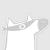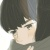0+0+0+0+0+0+0+0+0+0+0+0+0+0+0+0+0+0+0+0+0+0+0+0+Learning joint embedding space for various modalities is of vital importance for multimodal fusion. Mainstream modality fusion approaches fail to achieve this goal, leaving a modality gap which heavily affects cross-modal fusion. In this paper, we propose a novel adversarial encoder-decoder-classifier framework to learn a modality-invariant embedding space. Since the distributions of various modalities vary in nature, to reduce the modality gap, we translate the distributions of source modalities into that of target modality via their respective encoders using adversarial training. Furthermore, we exert additional constraints on embedding space by introducing reconstruction loss and classification loss. Then we fuse the encoded representations using hierarchical graph neural network which explicitly explores unimodal, bimodal and trimodal interactions in multi-stage. Our method achieves state-of-the-art performance on multiple datasets. Visualization of the learned embeddings suggests that the joint embedding space learned by our method is discriminative.0+0+0+下载预览0+0+0+0+0+0+0+0+0+As deep neural networks become more adept at traditional tasks, many of the most exciting new challenges concern multimodality---observations that combine diverse types, such as image and text. In this paper, we introduce a family of multimodal deep generative models derived from variational bounds on the evidence (data marginal likelihood). As part of our derivation we find that many previous multimodal variational autoencoders used objectives that do not correctly bound the joint marginal likelihood across modalities. We further generalize our objective to work with several types of deep generative model (VAE, GAN, and flow-based), and allow use of different model types for different modalities. We benchmark our models across many image, label, and text datasets, and find that our multimodal VAEs excel with and without weak supervision. Additional improvements come from use of GAN image models with VAE language models. Finally, we investigate the effect of language on learned image representations through a variety of downstream tasks, such as compositionally, bounding box prediction, and visual relation prediction. We find evidence that these image representations are more abstract and compositional than equivalent representations learned from only visual data.0+1+0+下载预览We study the problem of sampling from the power posterior distribution in Bayesian Gaussian mixture models, a robust version of the classical posterior. This power posterior is known to be non-log-concave and multi-modal, which leads to exponential mixing times for some standard MCMC algorithms. We introduce and study the Reflected Metropolis-Hastings Random Walk (RMRW) algorithm for sampling. For symmetric two-component Gaussian mixtures, we prove that its mixing time is bounded as $d^{1.5}(d + \Vert \theta_{0} \Vert^2)^{4.5}$ as long as the sample size $n$ is of the order $d (d + \Vert \theta_{0} \Vert^2)$. Notably, this result requires no conditions on the separation of the two means. En route to proving this bound, we establish some new results of possible independent interest that allow for combining Poincar\'{e} inequalities for conditional and marginal densities.0+0+0+下载预览It is necessary for clinicians to comprehensively analyze patient information from different sources. Medical image fusion is a promising approach to providing overall information from medical images of different modalities. However, existing medical image fusion approaches ignore the semantics of images, making the fused image difficult to understand. In this work, we propose a new evaluation index to measure the semantic loss of fused image, and put forward a Fusion W-Net (FW-Net) for multimodal medical image fusion. The experimental results are promising: the fused image generated by our approach greatly reduces the semantic information loss, and has better visual effects in contrast to five state-of-art approaches. Our approach and tool have great potential to be applied in the clinical setting.0+0+0+下载预览
Top